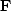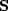Next: References Up: Adjoint operators Previous: Units

# STOLT MIGRATION

NMO is based on the quadratic equation v2 t2 = z2 + x2 (as explained in IEI). Stolt migration is based on the quadratic equation,which is the dispersion relation of the scalar wave equation. Stolt migration is NMO in the Fourier domain (see IEI). Denote the Fourier transform operator byand the Stolt operator by, where(32)

A property of matrix adjoints is.We know the transpose of NMO, and we know that the adjoint of Fourier transformation is inverse Fourier transformation. So(33)
We see then that the transpose to Stolt modeling is Stolt migration. (There are a few more details with Stolt's Jacobian.)Next: References Up: Adjoint operators Previous: Units
Stanford Exploration Project
10/21/1998